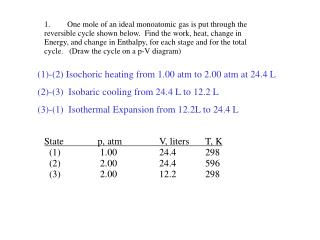DownloadDownload PresentationState p, atm V, liters T, K (1) 1.00 24.4 298 (2) 2.00 24.4 596 (3) 2.00 12.2 298

# State p, atm V, liters T, K (1) 1.00 24.4 298 (2) 2.00 24.4 596 (3) 2.00 12.2 298

Télécharger la présentation## State p, atm V, liters T, K (1) 1.00 24.4 298 (2) 2.00 24.4 596 (3) 2.00 12.2 298

- - - - - - - - - - - - - - - - - - - - - - - - - - - E N D - - - - - - - - - - - - - - - - - - - - - - - - - - -
##### Presentation Transcript

1. 1. One mole of an ideal monoatomic gas is put through the reversible cycle shown below. Find the work, heat, change in Energy, and change in Enthalpy, for each stage and for the total cycle. (Draw the cycle on a p-V diagram) (1)-(2) Isochoric heating from 1.00 atm to 2.00 atm at 24.4 L (2)-(3) Isobaric cooling from 24.4 L to 12.2 L (3)-(1) Isothermal Expansion from 12.2L to 24.4 L State p, atm V, liters T, K (1) 1.00 24.4 298 (2) 2.00 24.4 596 (3) 2.00 12.2 298

2. One mole of an ideal monoatomic gas CV = (3/2)R ; Cp= (5/2)R is put through the reversible cycle shown below. popp = p Find the work, heat, change in Energy, and change in Enthalpy for each stage and for the total cycle. Hnet = 0 Unet= 0 Cycle! (1)-(2) Isochoric heating from 1.00 atm to 2.00 atm at 24.4 L Isochoric: w=0 Ti = 298 Tf = 596 T = 298 U = CVT = 3.72 kJ H = CpT = 6.19 kJ qV = U = 3.72 kJ

3. (2)-(3) Isobaric cooling from 24.4 L to 12.2 L Isobaric: q = H = CpT Ti = 596 Tf = 298 T = -298 U = CVT = -3.72 kJ H = CpT = -6.19 kJ qp = H = -6.19 kJ w = -poppV = -2 (24.4-12.2) = 24.4 l.atm w = (8.314 J.mol-1.K -1 / 0.08205 l.atm mol-1.K -1) 22.4 l.atm w = 2.47 kJ = U - q

4. (3)-(1) Isothermal Expansion from 12.2L to 24.4 L Isothermal: H = 0 ; U = 0 w = -poppV = -RTln(Vf/Vi) = -1.72 kJ q = 1.72 kJ = - w q w U H 1-2 3.72 0 3.72 6.19 2-3 -6.19 2.47 -3.72 -6.19 3-1 1.72 -1.72 0 0 net -0.75 0.75 0 0

5. 2. Find an expression for the change in entropy when two blocks of the same substance and of equal mass, one at the temperature Thot and the other at Tcold are brought into thermal contact and allowed to reach equilibrium. Evaluate the change in temperature and Entropy for two 500-g blocks of copper assuming the molar heat capacity of Copper to be Cp = 24.4 J /(K mol) and taking Thot = 500K and Tcold = 250 K. Also, Determine the Entropy change of the Universe for this process.

6. 2. Find an expression for the change in entropy when two blocks of the same substance and of equal mass, one at the temperature Thot and the other at Tcold are brought into thermal contact and allowed to reach equilibrium. Evaluate the change in temperature and Entropy for two 500-g blocks of copper assuming the molar heat capacity of Copper to be Cp = 24.4 J /(K mol) and taking Thot = 500K and Tcold = 250 K. Also, Determine the Entropy change of the Universe for this process.

7. A gaseous sample consisting of 1.00 mol molecules is described by the equation of state • pV = RT(1 + Bp). • Initially at 373 K, it undergoes Joule-Thomson expansion from 100 atm to 1.00 atm. • Given that Cp = 5/2R • μJ-T = 0.21 K atm-1 • B = -0.525 (K/T) atm-1 • and that these are constant over the temperature range involved. • Calculate ΔT for the gas.

8. 3. A gaseous sample consisting of 1.00 mol molecules is described by the equation of state pV = RT(1 + Bp). Initially at 373 K, it undergoes Joule-Thomson expansion from 100 atm to 1.00 atm. Given that Cp = 5/2R μJ-T = 0.21 K atm-1 B = -0.525 (K/T) atm-1 and that these are constant over the temperature range involved, calculate ΔT for the gas.